# Slope of track

Calculate the average slope (in permille and even in degrees) of the rail tracks between Prievidza (309 m AMSL) and Nitrianske Pravno (354 m AMSL), if the track is 11 km long.

s1 =  4.09
s2 =  0.2344 °

### Step-by-step explanation:

${s}_{2}=\frac{180\mathrm{°}}{\pi }\cdot \mathrm{arctan}\left(\frac{y}{x}\right)=\frac{180\mathrm{°}}{\pi }\cdot \mathrm{arctan}\left(\frac{45}{10999.908}\right)=0.2344\text{°}=0\mathrm{°}1{4}^{\mathrm{\prime }}4\mathrm{"}$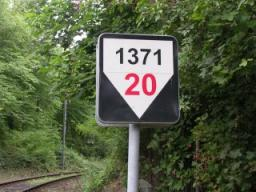Did you find an error or inaccuracy? Feel free to write us. Thank you!Tips to related online calculators
Do you want to convert length units?

#### You need to know the following knowledge to solve this word math problem:

We encourage you to watch this tutorial video on this math problem:

## Related math problems and questions:

• RiverCalculate how many permille river Dunaj average falls, if on section long 957 km flowing water from 1454 m AMSL to 101 m AMSL.Between cities A and B is route 13 km long of stúpanie average 7‰. Calculate the height difference of cities A and B.
• RailwayRailway line had on 5.8 km segment climb 9 permille. How many meters track ascent?
• Map - climb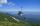On the map of High Tatras in scale 1:11000 are cable car stations in the Tatranska Lomnica and in the Skalnate Pleso with distance 354.6 mm. Altitude of this stations are 949 m and 1760 m. What is average angle of climb of this cable car track?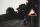A 5 km long road begins at an altitude 500 meters above sea level and ends at an altitude of 521 ASL. How many permille road rises?
• Slope of the pool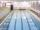Calculate slope (rise:run) of the bottom of the swimming pool long 30 m. Water depth at beginning of the pool is 1.13 m (for children) and depth at the end is 1.84 m (for swimmers). Slope express as percentage and as the angle in degrees.
• Cablecar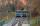Funicular on Petrin (Prague) was 408 meters long and overcomes the difference 106 meters in altitude. Calculate the angle of climb.
• Mountain railway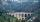Height difference between points A, B of railway line is 38.5 meters, their horizontal distance is 3.5 km. Determine average climb in permille up the track.
• Rail turn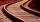The curve radius on the railway line is 532 m . Length of rail track on it is 818 m. How many degrees is the angle ASB, if A and B are boundary points and S is center of arc curve.What is the slope of a ladder 6.2 m long and 5.12 m in height.
• Height differenceWhat height difference is overcome if we pass a road 1 km long with a pitch21 per mille?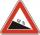The road sign informs the gradient is 10.9%. Calculate the angle at which the average decreases.The distance between the points P and Q was 356 m measured in the terrain. The PQ line can be seen from the viewer at a viewing angle of 107° 22 '. The observer's distance from P is 271 m. Determine the viewing angle of P and observer.From the observatory 11 m high and 24 m from the riverbank, river width appears in the visual angle φ = 13°. Calculate the width of the river.What percentage of the Earth’s surface is seen by an astronaut from a height of h = 350 km. Take the Earth as a sphere with the radius R = 6370 km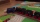A 160 m long passenger train runs on a double-track line in one direction at a constant speed of 54 km/h, and a 240 m long express train in the opposite direction. a) How fast is the express train if passing the passenger train driver for 6 s? b) How longThe height difference between points A and B is 475 m. Calculate the percentage of route climbing if the horizontal distance places A, B is 7.4 km.